Search IntMath
Close

450+ Math Lessons written by Math Professors and Teachers

5 Million+ Students Helped Each Year

1200+ Articles Written by Math Educators and Enthusiasts

Simplifying and Teaching Math for Over 23 Years

# 3. Division of Algebraic Expressions

Our first examples of division of algebraic expressions involve simplifying and canceling.

### Example 1

Simplify (3ab(4a^2b^5))/(8a^2b^3)

First, we multiply out the top line:

(12a^3b^6)/(8a^2b^3)

When we write it out in full, this means

(12 xx aaa xx b b b b b b)/(8 xx aa xx b b b)

Next, cancel the numbers top and bottom (we divide top and bottom by 4), the "a" terms (we cancel a^2=aa from top and bottom) and the "b" terms (we cancel b^3=b b b from top and bottom) to give us the final answer:

(3ab^3)/2

### Example 2

Simplify (12m^2n^3)/((6m^4n^5)^2)

We square the denominator (bottom) of the fraction:

(12m^2n^3)/((6m^4n^5)^2)=(12m^2n^3)/(36m^8n^10)

Next, we cancel out the numbers, and the "m" and "n" terms to give the final answer:

1/(3m^6n^7)

### Example 3

Simplify (6p^3q^2-10p^2q)/(4q)

With this example, we'll break it into 2 fractions, both with denominator 4q to make it easier to see what to do.

(6p^3q^2-10p^2q)/(4q)=(6p^3q^2)/(4q)-(10p^2q)/(4q)

Next, we cancel the numbers and variables:

(3p^3q)/2-(5p^2)/2

Finally, we combine the fractions:

(3p^3q-5p^2)/2

After you have had some practice with these, you'll be able to do it without separating them into 2 fractions first.

## Dividing by a Fraction

Recall the following when dividing algebraic expressions.

The reciprocal of a number x, is 1/x.

For example, the reciprocal of 5 is 1/5 and the reciprocal of 1 2/3 is 3/5.

To divide by a fraction, you multiply by the reciprocal of the fraction.

For example, 3/4 -: 7/x=3/4xxx/7=(3x)/28

### Example 4

Simplify

(3+1/x)/(5/x+4)

I'll show you how to do this two different ways. It is worth seeing both, because they are both useful. You can decide which is easier ;-)

#### Solution 1 - Multiplying by the Reciprocal

I take the top expression (numerator) and turn it into a single fraction with denominator x.

3+1/x=(3x+1)/x

We do likewise with the bottom expression (denominator):

5/x+4=(5+4x)/x

So the question has become:

(3+1/x)/(5/x+4)=((3x+1)/x)/((5+4x)/x)

We think of the right side as a division of the top by the bottom:

(3x+1)/x-:(5+4x)/x

To divide by a fraction, you multiply by the reciprocal:

(3x+1)/(x)xxx/(5+4x)=(3x+1)/(5+4x)

The x's cancelled out, and we have our final answer, which cannot be simplified any more.

#### Solution 2 - Multiplying Top and Bottom

I recognise that I have "/x" in both the numerator and denominator. So if I just multiply top and bottom by x, it will simplify everything by removing the fractions on top and bottom.

(3+1/x)/(5/x+4)xxx/x

I am really just multiplying by "1" and not changing the original value of the fraction - just changing its form.

So I multiply each element of the top by x and each element of the bottom by x and I get:

(3+1/x)/(5/x+4)xxx/x=(3x+1)/(5+4x)

I cannot simplify any further.

## Long Division in Algebra

Before we do an example using algebra, let’s remember how to do long division with numbers first.

### Example 5

Let’s do 23,576 divided by 13.

We can write this as a fraction:

23576/13

Now, to divide this, (assuming we do not have a calculator) we could proceed as follows.

23 divided by 13 = 1 with remainder 10.

We bring the 5 (the next number after 3) down.

Now we have

105 divided by 13 is 8 with remainder 1

We continue until we get to the last number, 6.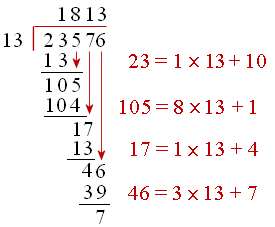Our result means that the answer is 1,813 with remainder 7, or:

23576/13=1813 7/13

We use a similar technique for long division in algebra.

### Example 6 - Algebraic Long Division

Simplify (3x^2-11x-4)-:(x-4)

We are dividing a polynomial of degree 2 by a polynomial of degree 1. This is algebraic long division.

Step 1: We look at the first term of (3x2 − 11x − 4) and the first term of (x − 4).

Divide as follows: 3x2 ÷ x = 3x

We write 3x at top of our long division and multiply (3x)(x − 4) = 3x2 − 12x to give the second row of our solution.Step 2: Subtracting the second row from the first gives:Be careful with

-11x − (-12x) = -11x + 12x = x

Step 3: Bring down the -4 from the first row: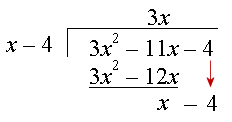Step 4: Divide x (in the 3rd row) by x from the (x − 4) in the question. Our answer is 1 and we write "+ 1" at the top of our long division.

Next, multiply (1) by (x − 4) to get the 4th row.Step 5: Subtract the 4th row from the 3rd:So (3x2 − 11x − 4) ÷ (x − 4) = 3x + 1

You can check your answer by multiplying (3x + 1) by (x − 4) and you'll get (3x2 − 11x − 4).

### Example 7

Simplify (6x^2+6+7x)/(2x+1)

We can think of (6x^2+6+7x)/(2x+1) as (6x2 + 7x + 6) ÷ (2x + 1)

Once again we are dividing a polynomial of degree 2 by a polynomial of lower degree (1). This is algebraic long division.

Step 1: 6x2 ÷ 2x = 3x

So we write the following, using (3x)(2x + 1) = 6x2 + 3x for the second row: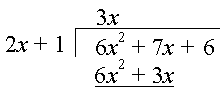Step 2: We subtract 6x2 + 3x from the first row: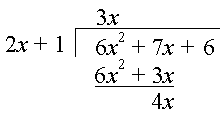Step 3: Bring down the 6: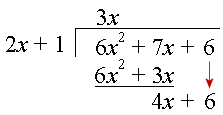Step 4: Divide 4x by 2x. Our answer is 2 and we multiply 2(2x + 1) to get the 4th row.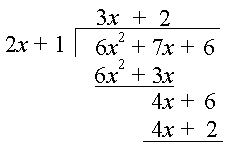Step 5: Subtract, and we are left with 4.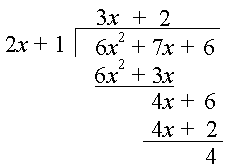(6x^2+6+7x)/(2x+1)=3x+2+4/(2x+1)

NOTE: Some people prefer to write the problem with all the x2's, x's and units in line, as follows: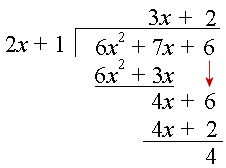You can see how algebraic long division is used in a later section, Remainder and Factor Theorems.

## Problem SolverThis tool combines the power of mathematical computation engine that excels at solving mathematical formulas with the power of GPT large language models to parse and generate natural language. This creates math problem solver thats more accurate than ChatGPT, more flexible than a calculator, and faster answers than a human tutor. Learn More.